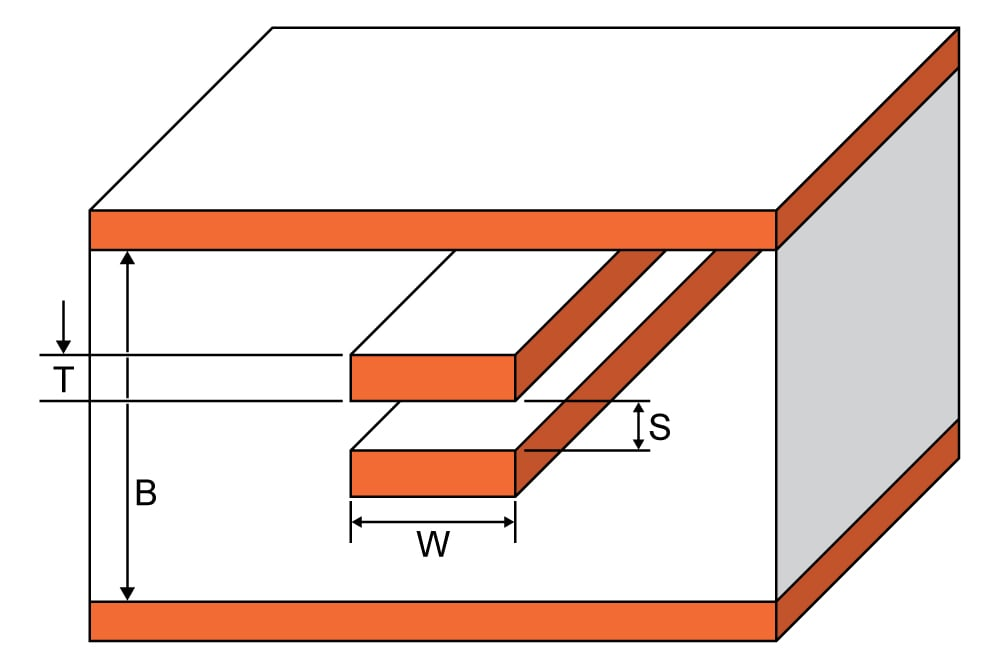# Broadside Coupled Stripline Impedance Calculator

## This calculator helps you compute the characteristic impedance of a broadside coupled stripline.

Ohms
Ohms
Ohms
Ohms

### Overview

This calculator is designed to calculate the characteristic impedance of a broadside couple stripline (see diagram below). The required values are trace thickness, substrate heights, trace width, and subtrate dielectric in the calculator above and press the "calculate" button. The default units for all given values, except the subtrate dielectric, is in mil. It is possible to select other units.### Equations

$$Z_{0} = \frac{80}{\sqrt{\epsilon_{r}}}ln\left(\frac{1.9(2H+T)}{0.8W+T}\right)\left(1-\frac{H}{4(H+S+T)}\right)$$

Where:

$$H = B - \frac{(S+T)}{2}$$

$$T$$ = trace thickness

$$W$$ = trace width

$$S$$ = trace spacing

$$B$$ = substrate height

$$\epsilon_{r}$$ = relative permittivity

### Notes:

Odd Impedance ($$Z_{0_{odd}}$$): The impedance between one differential trace and the ground plane with the differential signals are driven with opposite polarity.

$$Z_{0_{odd}}=\frac{Z_{0_{diff}}}{2}$$

Even Impedance ($$Z_{0_{even}}$$): The impedance between one differential trace and the ground plane with the differential signals are driven with the same signal.

$$Z_{0_{even}} = 2Z_{0_{common}}$$

Differential Impedance ($$Z_{0_{diff}}$$): The impedance between the two lines with opposite polarity signals.

$$Z_{0_{diff}} = 2Z_{0_{odd}}$$

Common Impedance ($$Z_{0_{common}}$$):The impedance between the two lines with the same signal.

$$Z_{0_{common}}=\frac{Z_{0_{even}}}{2}$$

### Applications

Broadside-coupled striplines commonly comes with two substrate dielectrics. Although this is apparently a simple construction, the fact is that it is actually a pain to fabricate to produce consistent characteristic impedance. The difficulty rises from both inner-layer misregistration and slight offsets and differences in etching. This is particularly true for fine-line traces. Despite having internal layers with less effort on processing, the most common structure for the broadside-coupled stripline is that with both traces overlaid for maximum coupling.